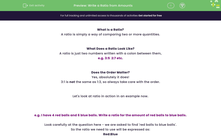# Write a Ratio from Amounts

In this worksheet, students will practise creating ratios to compare two quantities which have something in common. They will express these ratios in the form a:b, but will not be asked to simplify at this point.Key stage:  KS 4

Year:  GCSE

GCSE Subjects:   Maths

GCSE Boards:   AQA, Eduqas, Pearson Edexcel, OCR,

Curriculum topic:   Ratio, Proportion and Rates of Change

Curriculum subtopic:   Ratio, Proportion and Rates of Change Calculations with Ratio

Difficulty level:#### Worksheet Overview

What Is a Ratio?

A ratio is simply a way of comparing two or more quantities.

What Does a Ratio Look Like?

A ratio is just two numbers written with a colon between them,

e.g. 3:5  2:7 etc.

Does the Order Matter?

Yes, absolutely it does!

3:1 is not the same as 1:3, so always take care with the order.

Let's look at ratio in action in an example now.

e.g. I have 4 red balls and 6 blue balls. Write a ratio for the amount of red balls to blue balls.

Look carefully at the question here - we are asked to find 'red balls to blue balls'.

So the ratio we need to use will be expressed as:

Red:Blue

Now we just need to substitute the correct numbers into this ratio:

4:6

While this ratio can be cancelled down, don't worry about that at this point, we will focus on learning how to do this in the Level 2 activity on ratio.

In this activity, we will create ratios to compare two quantities which have something in common. We will express these ratios in the form a:b, but we will not be simplifying them at this point.

### What is EdPlace?

We're your National Curriculum aligned online education content provider helping each child succeed in English, maths and science from year 1 to GCSE. With an EdPlace account you’ll be able to track and measure progress, helping each child achieve their best. We build confidence and attainment by personalising each child’s learning at a level that suits them.

Get started